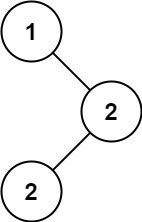### LeetCode• ㊗️
• 大家
• offer
• 多多！

## Problem

Given the root of a binary search tree (BST) with duplicates, return all the mode(s) (i.e., the most frequently occurred element) in it.

If the tree has more than one mode, return them in any order.

Assume a BST is defined as follows:

• The left subtree of a node contains only nodes with keys less than or equal to the node’s key.
• The right subtree of a node contains only nodes with keys greater than or equal to the node’s key.
• Both the left and right subtrees must also be binary search trees.

Example 1:Input: root = [1,null,2,2]
Output: 


Example 2:

Input: root = 
Output: 


## Code

class Solution {
int currVal;
int currCount = 0;
int maxCount = 0;
int modeCount = 0;
int[] modes;

public int[] findMode(TreeNode root) {
inorder(root);

modes = new int[modeCount];
modeCount = 0;
currCount = 0;

inorder(root);

return modes;
}

private void inorder(TreeNode root) {
if (root == null) return;

inorder(root.left);
handleValue(root.val);
inorder(root.right);
}

private void handleValue(int val) {
if (val != currVal) {
currVal = val;
currCount = 0;
}

currCount++;

if (currCount > maxCount) {
maxCount = currCount;
modeCount = 1;
} else if (currCount == maxCount) {
if (modes != null) {
modes[modeCount] = currVal;
}

modeCount++;
}
}
}